# Theory 1: The gradient equation

Abstract In today’s world, gradient elution is used more and more as a generally useful technique, supplanting isocratic chromatography in many applications. It is of course most valuable in generic applications, where the content of the sample is unknown. In addition, it becomes inevitable for samples with a wide range of retention properties.

In this chapter, I will cover the theoretical aspects of gradient chromatography, starting with a thorough treatment of linear elution theory.

While the linear elution theory is more generally applicable, the focus here will be on reversed-phase chromatography. After the definitions of the variants of peak capacity in gradients, I will examine the maximization of the peak capacity for specific analytical problems. Finally, I will briefly discuss the phenomenon of peak compression, which enhances the resolving power of gradient chromatography.

## Effect of gradient on retention

In this section, we will occupy ourselves with the fundamentals of the gradient equation. A more in-depth analysis can be found in textbooks, especially in reference . The treatment here is a subtle simplification of Snyder’ treatment, but the elements are very similar.

In a gradient, the retention factor k of a compound is constantly changing with the mobile phase composition (see Sidebar 1 for more detailed equations). The dependence of the retention factor on the solvent composition c can often be described by a simple log-linear relationship: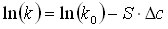(1)

k0 is the retention factor in a reference composition, in our case here in the solvent composition at the beginning of the gradient, and S is the slope of this relationship. ∆c is the difference in the solvent composition between the reference state with the retention factor k0 and the composition that delivers the retention factor k. The slope S depends on the molecular weight of the analyte. Its value increases roughly with the square root of the molecular weight of the analyte.

While such a simple linear relationship is not accurate for a very wide range of solvent compositions , it is definitely a suitable description for the range of solvent compositions in which the peak is actually moving through the column (as compared to the time when it “sits” at the column inlet with little migration). If we then change the solvent composition linearly with time, the natural logarithm of the retention factor will then also change linearly with time. This permits a simple integration and derivation of the retention of an analyte as a function of the way we carry out the gradient [1, 3].

## Equation for the retention factor

Click here to see in the sidebar how we come to the following formula. The derivation shown in the sidebar results in the following equation for the retention factor under gradient conditions kg(2)

We have used the following abbreviations in this equation: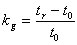(3a) and(3b)

G is the generalized gradient slope, comprising the slope S of equation 1, the difference in the solvent composition from the beginning to the end of the gradient ∆c, and the inverse ratio of the gradient run time tg to the column dead time t0. To complete the information, we also need to know the peak width under gradient conditions, which is primarily determined by the retention factor at the point of elution ke. This retention factor is calculated by simply introducing the gradient retention factor into the equation S1.3:(4a)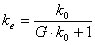(4b)

Equations 2 and equation 4b are a complete description of the behavior of a peak under gradient conditions using the linear solvent strength model . The gradient parameter G from equation 3b contains all the elements of the gradient separation: the slope S of the relationship between the natural logarithm of the retention factor and the solvent composition, which is a property of the analyte, the column and the mobile phase chosen, and the experimental gradient slope  that is open to the manipulation of the investigator.

## Example 1: effect of changing the column

This is discussed in more detail in the section on gradient scaling.

Conversely, changing the gradient run time alone, while keeping a constant difference in solvent composition from the beginning to the end of the gradient is the first tool recommended for changing the selectivity of a separation . Now the value of the gradient slope changes for all compounds, and the resolution will change. If the value of S varies randomly throughout the chromatogram, many individual changes in the selectivity can be observed. If the value of S changes in a regular pattern, such as for a group of oligomers, where S increases with the molecular weight of the analyte, then easily predictable changes in the elution pattern occur with a change in the gradient slope. A good rule of thumb is that the slope S changes with the square root of the molecular weight of the analytes.

The same changes in retention and selectivity can be achieved by changing the flow rate, while keeping the gradient run time constant. While this is not common practice, it is a simple approach to decrease the run time required during method development. A limitation to this approach can be the pressure limit of the instrument, or to a much lesser degree, the deterioration in plate count. It needs to be pointed out clearly that this situation is completely different from isocratic chromatography, where retention times and peak widths change with the flow rate, while the selectivity of the separation remains constant.

## Example 2: increasing run time

(Figure 2: Change in retention with an expansion of the gradient. Top: 10 minute gradient. Bottom: 30 minute gradient. (Click to open pdf))

In these examples in figure 1 and 2, we have seen the three possibilities of changes in the elution pattern with changing gradients:

1. The analytes have the same slope S and their retention changes in parallel. In this case, the resolution improves as the gradient expands (dotted lines).
2. Two analytes with a different slope S elute close to each other, and a change in the gradient steepness will affect their resolution and relative position (compounds 5 and 6).
3. A group of analytes have slopes S that increase with the retention factor at the beginning of the gradient (solid lines). These compounds will show a synchronized shift in the gradient elution pattern as the gradient steepness is changed (example: oligomers).

The elution pattern in a gradient also depends on the initial conditions. This is less pronounced, when large molecules like peptides are the analytes. Also, for a given gradient slope, the later eluting compounds are less affected than the early eluting compounds, or even do not change their relative positions at all. Nevertheless, the starting conditions to the gradient are an additional tool in method development especially for the early eluting analytes.

## Example 3: changing the initial conditions

The elution pattern in a gradient also depends on the initial conditions. This is less pronounced, when large molecules like peptides are the analytes. Also, for a given gradient slope, the later eluting compounds are less affected than the early eluting compounds, or even do not change their relative positions at all. Nevertheless, the starting conditions to the gradient are an additional tool in method development especially for the early eluting analytes.

Figure 3 shows an example of shifts in retention for a fairly complex sample containing 20 analytes. The separation at the bottom was completely optimized in a simulation for a high-performance UPLC column (N ~ 25 000) and contains all 20 peaks. The gradient at the bottom was executed with a gradient slope of 50% over 22 minutes with a starting composition of 20% organic. The gradient at the top started with 0% organic with the same gradient slope.  One can see that the retention pattern at the end of the chromatogram did not change, while more significant changes in peak position and elution order are observed early in the chromatogram. Specifically, the small peak indicated by the solid line coeluted with its neighbor in the top chromatogram.

(Figure 3: Changes in the elution pattern due to changes in the initial condition of gradients with the same gradient slope. )

In general, or on average, resolution in gradient chromatography can be treated in the same way as in isocratic chromatography [1, 4]. In isocratic chromatography, the resolution equation looks as follows: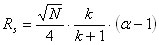(5)

with the usual definitions: N is the plate count, k is the retention factor, and α is the relative retention between the two closely eluting compounds under consideration. If α does not change with retention, one can consider the influence of these three parameters on the resolution separately.

A very similar equation can be derived for gradients [1, 4]: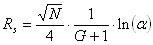(6)

α is the same relative retention as considered for isocratic chromatography above. The derivation included a simplification of equation 4b for a large

k0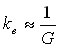(7)

and the similarity increases further: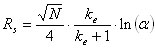(8)

As above for the resolution equation for isocratic chromatography, if α does not change with retention, the resolution increases with an increase in ke, the retention factor at the point of elution. Since ke always increases with a flattening of the gradient, the resolution generally increases under these conditions, as pointed out above. However, as in isocratic chromatography, this may not be true for a specific pair of analytes, due to the specific changes in the elution order with solvent composition. Only the average resolution increases with a flattening of the gradient.